# Prediction of Transit Time on Urban Roads Based on Particle Filtering

Prediction of Transit Time on Urban Roads Based on Particle Filtering
College of Electrical and Information Engineering, Quzhou University, Quzhou 324000, China

Corresponding Author Email:
chaig@qzc.edu.cn
Page:
189-194
|
DOI:
https://doi.org/10.18280/ria.340209
10 December 2019
|
Accepted:
21 February 2020
|
Published:
10 May 2020
| Citation

OPEN ACCESS

Abstract:

The transit time on roads is essential to the intelligent traffic system in urban areas. If the transit time is predicted accurately, the traffic management system can work more effectively, and the public will enjoy rational travel strategies. However, the traditional prediction algorithms for transit time cannot adapt well to the complex road network and the sparsely deployed sensors in cities. By contrast, the particle filtering (PF) algorithm has strong adaptability to such a stochastic nonlinear problem. Therefore, this paper measures the spatiotemporal similarity between historical data on different roads at different moments with speed matrices, aiming to prevent the data degradation in transit time prediction. Besides, the PF algorithm was innovatively applied to build a transit time prediction model on urban roads. The traffic trend in historical data was modelled with weighted particles. Finally, the effectiveness of our algorithm was demonstrated through an empirical analysis. The results show that our algorithm outperforms the other transit time prediction algorithms in prediction accuracy and computing performance.

Keywords:

urban roads, transit time prediction, particle filtering, traffic network, speed matrix

1. Introduction

The prediction accuracy of urban road transit time determines the efficiency of urban traffic management, playing an important role traffic management and planning [1, 2]. If the transit time is predicted accurately, the travelers will access the correct information on road traffic. This enables the drivers to optimize their travel strategies in time, and circumvent the congested roads. Being a performance index of the traffic system, the transit time also facilitates the effectiveness comparison between different traffic management strategies in a quantitative manner. Hence, it is an effective way to enhance the efficiency of traffic management system by accurately predicting the road transit time.

The existing prediction methods for road transit time are either driven by data or based on models. The data-driven methods forecast the transit time by mining the relationship between historical and current traffic modes. This type of methods only examines the traffic modes reflected by the traffic data, instead of depicting the internal properties of the traffic flow or setting up the state transfer function between traffic states of adjacent moments. If classified by theories and principles , the methods driven by data are either parametric and nonparameteric [4-13]. The classification of the data-driven methods is illustrated in Figure 1 below.

Most of the model-based methods predict road transit time in three steps: building a dynamic macro model of the traffic network, calibrating the model parameters with historical data, and forecasting future transit time by the calibrated model. Early model-based prediction methods for road transit time are all grounded on dynamic models of the traffic network on the marco, meso and micro scales. The assumptions of these methods are too ideal to demonstrate the effects of various factors on road transit time in actual traffic environment.

With the development of computer technology, the AI algorithm based on particle filtering (PF), has been introduced to predict road transit time [14-16]. These algorithms enjoy two unique advantages: First, the state transfer function can be obtained accurately from macro traffic model, revealing the relationship between traffic states of adjacent moments; the state transfer function thus obtained manifests the inherent physical law of traffic flow, and overcomes a major defect of the state transfer function derived from historical data, namely, the interference from the noises in historical data. Second, the measurement and time are updated simultaneously at each moment under the Bayesian filtering model; once obtained, a measured value is used to make a new estimation, and derive a new prediction via the state transfer function.

The PF-based AI algorithm outperforms the methods that directly utilize historical data in transit time prediction. However, the assumption that the noises are distributed normally contradicts the complex scenarios in the real world. This problem can be resolved by the PF, a sequential Monte-Carlo (MC) method. The PF can solve dynamic problems with strong nonlinearity, without needing the above assumption. Over the years, fruitful results have been achieved in transit time prediction with the PF and nonlinear state transfer function [17-21]. The urban traffic network is highly nonlinear, making the PF the best prediction strategy for transit time on urban roads. However, most PF algorithms are designed for transit time prediction on expressways, which is much easier than that on urban roads. It is an arduous task to predict the transit time on urban roads in an accurate manner. For one thing, the traffic data in urban traffic network are not suitable, due to the complex traffic environment in urban areas, as well as the diversity and sparsity of the data collected by probe vehicles; for another, the traffic lights and intersections on urban roads pose severe challenges to traffic flow modelling and estimation .

## 1.jpgFigure 1. The classification of the data-driven methods

To sum up, the existing prediction methods for transit time each has its limitations and defects on the forecast of urban road transit time. Therefore, this paper adopts the speed matrix to measure the spatiotemporal similarity of historical data of different road sections at different moments, preventing data degradation in transit time prediction. Besides, the PF algorithm was innovatively applied to build a prediction model of transit time on urban roads. The traffic trend in historical data was modelled with weighted particles. Finally, the prediction accuracy and adaptability of the proposed approach were tested through empirical analysis.

The remainder of this paper is organized as follows: Section 2 establishes a prediction model of transit time on urban roads based on the PF algorithm, considering the complex features of urban road network, and fully explains the basic principles of the algorithm; Section 3 verifies the proposed model through case analysis, and confirms the effectiveness of the PF algorithm through the selection of model parameters and comparison with two regular model-based methods; Section 4 puts forward the conclusions of this research.

2. Methodology

Under the complex scenarios of urban roads, the strong nonlinearity of traffic data and non-Gaussian distribution of noises must be considered in transit time prediction. Compared with other algorithms, the PF algorithm can easily handle stochastic nonlinear problems with non-Gaussian distribution. Therefore, this paper takes the speed matrix of each urban road as a particle, and applies the PF algorithm to predict the transit time on urban roads, aiming to achieve accurate predictions under complex scenarios.

2.1 Basic principles of the PF algorithm

## 2.jpg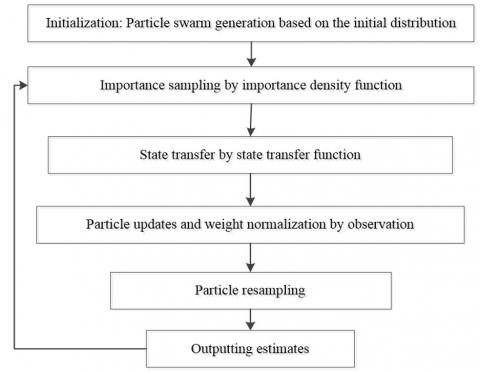Figure 2. The standard flow of the PF algorithm

Proposed by Gordon in 1993, the PF algorithm mainly relies on the MC method to calculate the posterior probability density of random samples, and thus obtain the quasi-optimal solution to physical models. The PF algorithm (Figure 2) can be implemented through initialization, particle weight update, and importance sampling.

2.2 PF-based prediction of transit time

To reflect the spatiotemporal features of urban traffic network, this paper treats the speed matrix of each road as a particle for transit time prediction. The speed matrix M is a tool to characterize the spatiotemporal features of traffic flow. The matrix contains the speed on each road in the set of roads R={r1, r2, …, rm} at every moment in the set of moments T={t1, t2, …, tn}. Every element Vi, j in the matrix M describes the speed on road i at moment j. Figure 3 gives an example of speed matrix.

## 3.jpg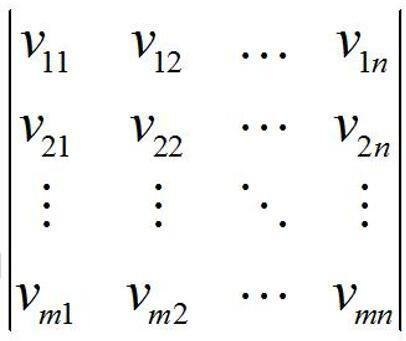Figure 3. An example of speed matrix M

In the PF algorithm, the set of roads R contains the target road r and any other road whose distance from r is no greater than 2; the set of moments T covers the current moment t and the previous p-1 moments, i.e. T={t-p+1, t-p+2, …., t-1, t}. For convenience, the roads whose distance from r is 1 are called one-hop roads, and the roads whose distance from r is 2 are called two-hop roads.

According to the definition of the speed matrix, this paper models the similarity between different traffic modes at different moments. Firstly, the particles were initialized by randomly generating speed matrices. Next, the particle weights were updated by the weight formula. Further, the necessity of resampling was determined by evaluating the degree of degradation. The particles failing to satisfy the threshold were resampled by random importance resampling algorithm. Finally, the weighted mean of all effective particles was taken as the prediction result, completing the forecast of transit time on complex urban roads. The workflow of the PF-based prediction of transit time are as follows:

Step 1. Particle initialization

Taking the speed matrix on each road as a particle, particle initialization is equivalent to setting up the initial speed matrices. Suppose the target road r and other n-1 roads belong to the same set R, and the initial speed matrices are generated randomly at moment $t_{0}^{i}$. Then, an initial speed matrix $M_{0}^{i}$ can be expressed as:

## 4.jpg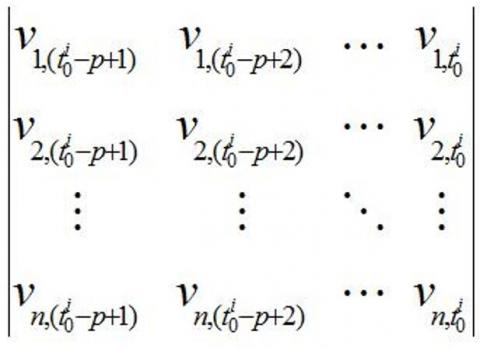Figure 4. An initial speed matrix $M_{0}^{i}$

In our PF algorithm, each particle represents a speed matrix. The similarity between speed matrices determines the value of particle weights. Hence, the particle weights were updated based on the similarity between speed matrices. The weight of particle i can be updated by:

$w_{t}^{i}=\mathrm{w}_{t-1}^{i} *\left[\rho\left(m_{1}^{i}, m_{1}^{c}\right)+\frac{\lambda_{1}}{n_{1}} \sum_{j=1}^{n_{1}} \rho\left(m_{1+j}^{i}, m_{1+j}^{c}\right)\right.$

$\left.+\frac{\lambda_{2}}{n_{2}} \sum_{j=1}^{n_{2}} \rho\left(m_{1+n_{1}+j}^{i}, m_{1+n_{1}+j}^{c}\right)\right]$          (1)

where, $M_{t}^{i}$ and $M_{t}^{c}$ are the speed matrices for the traffic mode of particle i and that of current traffic mode at moment t, respectively; mi is the data on the i-th row of a speed matrix; n1 and n2 are the number of one-hop and two-hop roads, respectively; ρ is the linear correlation coefficient between the element of a road in $M_{t}^{i}$ and that in $M_{t}^{c} ; \lambda_{1}$ and $\lambda_{2}$ are the weights of one-hop and two-hop roads, respectively.

Step 3. Importance resampling

Before importance resampling, the necessity of resampling was determined by evaluating the degree of degradation of each particle. Let Neff be the number of effective particles, and $W_{t}^{i}$ be the weight of particle I at moment t. Then, the degree of degradation was assessed by the relative numerical efficiency (RNE) proposed by Ren et al. :

$N_{e f f}=\frac{1}{\sum_{i=1}^{N}\left(w_{t}^{i}\right)^{2}}$          (2)

Let Nth be the threshold of the number of effective samples. If NeffNth, go to Step 4; if NeffNth, conduct importance resampling by random.

In the random importance resampling method, the length of the sampling interval depends on the corresponding importance. The length was calculated based on the similarity between the historical data and the current day data. For each particle to be resampled, a random value $\sigma^{i} \in\left[0, c^{D}\right]$ was generated within its sampling interval, and used to create a new speed matrix for transit time prediction. In this way, the interval length can be determined with the traffic mode the most similar to that represented by historical data.

Step 4. Transit time prediction

Through the above steps, the speed matrices at multiple sampling moments were obtained. Then, the data of all particles were moved along the time window by P moments, without changing the weight of any particle. The weighted mean of all particles was taken as the prediction result. In other words, the transit time $\overline{x_{t+p}}$ can be predicted by:

$\overline{x_{t+p}}=\frac{\sum_{i=1}^{N} w_{t}^{i} x_{t+p}^{i}}{\sum_{i=1}^{N} w_{t}^{i}}$          (3)

where, wit is the weight of particle i; xit+p is the transit time after moment p.

3. Example Analysis

This section aims to verify our PF algorithm with the dataset collected by a real probe vehicle in a region [24, 25]. In the dataset, each frame of data consists of the ID, coordinates of a vehicle, and time. Based on the regional dataset, the data on typical congested urban roads were selected as the basic data to verify the algorithm.

3.1 Parameter selection

To disclose how different parameters affect the prediction, the errors of our PF algorithm were analyzed by changing one of the following three parameters under different forecast periods (10, 20, 30, 40, 50 and 60min): data length, number of particles and resampling ratio.

Figures 5-7 provide the mean percentage absolute errors (MAPEs) of our PF algorithm, with the data length p increasing from 1 to 10, the number of particles N growing from 0 to 400, and the resampling ratio rising from 0.1 to 0.9, respectively.

## 5.jpg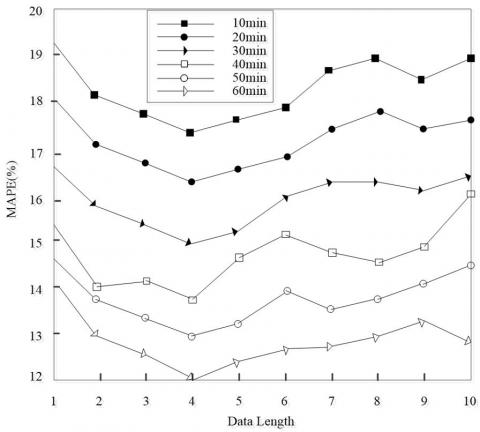Figure 5. Relationship between algorithm error and data length

## 6.jpg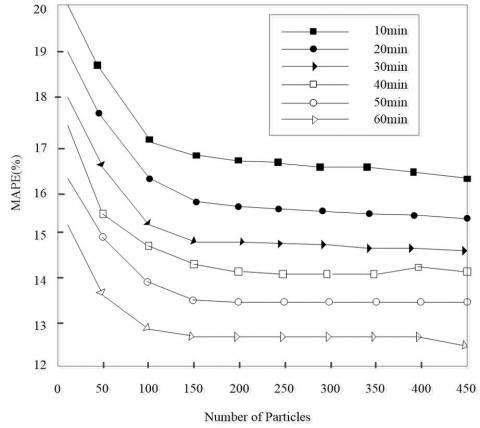Figure 6. Relationship between algorithm error and the number of particles

From Figures 5-7, it can be seen that, with the extension of the forecast period, the algorithm error was steadily on the rise. Thus, the prediction is more accurate under a short forecast period, which agrees with the views of relevant research.

As shown in Figure 5, the algorithm error first decreased and then increased, with the growth in data length. The minimum MAPE was achieved at the data length of 4. Thus, the optimal data length was set to 4.

As shown in Figure 6, the algorithm error continued to drop, as the number of particles grew from 0 to 100. With further growth in the number of particles, however, the algorithm error declined at an increasingly slow speed and basically remained the same. Therefore, the optimal number of particles was determined as 100.

## 7.jpgFigure 7. Relationship between algorithm error and resampling ratio

As shown in Figure 7, the algorithm error had a wavelike increase, with the growth in resampling ratio. The MAPE reached the minimum at the resampling ratio of 20%, which was thus taken as the optimal resampling ratio.

In the light of the above, the optimal parameters for algorithm verification were configured as follows: the data length in speed matrices p=4; the number of particles N=100; the resampling ratio of particles, 20% (i.e. the number of effective particles Nval=80).

3.2 Algorithm verification

To verify its prediction effect of transit time on urban roads, our PF algorithm was compared with two popular algorithms in transit time prediction, namely, the Kalman filtering (KF) algorithm and the nearest neighbor (NN) algorithm.

To predict the transit time, the KF algorithm mainly adopts the ratio between measured values at adjacent moments as the state transfer function, and models the transit time prediction as a linear system problem. Similarly, the NN algorithm is a mainstream strategy to solve linear problems. This algorithm searches for the n historical data most similar to the current traffic mode, and takes the weighted mean of these data as the prediction result.

Let N be the total number of samples. The mean absolute error (MAE) and the MAPE were calculated to compare the effects of the three algorithms:

$M A E=\frac{1}{N} \sum_{i=1}^{N}\left|\bar{T}-T_{i}\right|$          (4)

$M A P E=\frac{1}{N} \sum_{i=1}^{N}\left|\frac{\bar{T}-T_{i}}{\bar{T}}\right| * 100 \%$          (5)

where, Ti and $\bar{T}$ are the i-th predicted value, and the true value, respectively.

Next, the three algorithms were applied under different forecast periods, with p=4, N=100 and Nval=80. The prediction errors of them are compared in Figure 7.

## 8.jpg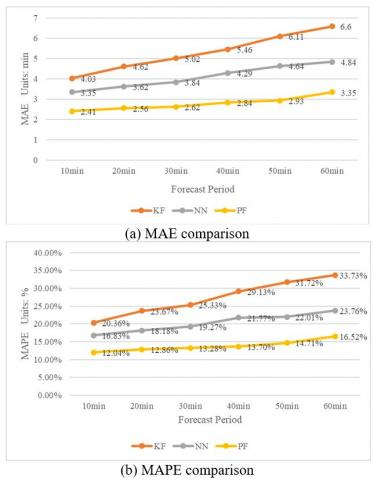Figure 8. Comparison of prediction results

As shown in Figure 8, our PF algorithm achieved less error than the KF algorithm and the NN algorithm, in both MAE and MAPE. This means the prediction accuracy of transit time is greatly enhanced by introducing the speed matrices of target roads to the PF algorithm.

With different prediction accuracies, the three algorithms all exhibited a growing trend in both MAE and MAPE, i.e. a gradual decline in prediction accuracy. Thus, our algorithm only improves the prediction accuracy to a certain extent, failing to fully eliminate the impact of long forecast time on prediction error.

Despite achieving the highest accuracy and lowest error in transit time prediction on urban roads, our PF algorithm requires a strong computing power and consumes a long computing time. There is still ample room for improvement before realizing real-time prediction of transit time on urban roads.

On the contrary, the KF algorithm and the NN algorithm may not be able to achieve high prediction accuracy as our algorithm, but these two classic algorithms boast a high computing efficiency. The NN algorithm outshines similar methods in real-timeliness and stability of prediction, while controlling the prediction errors in the allowable range. That is why this algorithm is widely used in traffic control.

4. Conclusions

The transit time prediction of urban road network has long been a hot topic in intelligent traffic control. Considering the typical features of congested urban roads, this paper establishes a transit time prediction model based on the PF algorithm. The speed matrix of each target road was taken as a particle, and the initial speed matrices were used to represent the traffic mode at a moment of the historical traffic data. In addition, the weight coefficients were introduced to promote the real-time performance of the prediction. The particles with a low weight were subjected to importance resampling to prevent the degradation problem of traditional algorithms. Through example analysis, our algorithm was proved to outperform the traditional KF algorithm and NN algorithm in the accuracy and stability of transit time prediction on urban roads. Our PF algorithm can improve the prediction accuracy of transit time on urban roads to a certain extent. However, the computing efficiency should be further improved to meet the real-time requirements on intelligent control of urban traffic.

Acknowledgment

The Project Supported by Zhejiang Provincial Natural Science Foundation of China (LQ17F030005).

References

 Wang, J., Indra-Payoong, N., Sumalee, A., Panwai, S. (2013). Vehicle reidentification with self-adaptive time windows for real-time travel time estimation. IEEE Transactions on Intelligent Transportation Systems, 15(2): 540-552. https://doi.org/10.1109/TITS.2013.2282163

 Chen, H., Rakha, H.A. (2014). Real-time travel time prediction using particle filtering with a non-explicit state-transition model. Transportation Research Part C: Emerging Technologies, 43: 112-126. https://doi.org/10.1016/j.trc.2014.02.008

 Oh, S., Byon, Y.J., Jang, K., Yeo, H. (2017). Short-term travel-time prediction on highway: A review of the data-driven approach. Transport Reviews, 35(1): 4-32. https://doi.org/10.1080/01441647.2014.992496

 Kwon, J, Coifman, B, Bickel, P. (2000). Day-to-day travel-time trends and travel-time prediction from loop-detector data. Transportation Research Record, 1717(1): 120-129. https://doi.org/10.3141/1717-15

 Zhang, X., Rice, J.A. (2003). Short-term travel time prediction. Transportation Research Part C: Emerging Technologies, 11(3-4): 187-210. https://doi.org/10.1016/S0968-090X(03)00026-3

 Zhang, J.H., Zhu, Q., Song, L. (2019). Self-adaptive hierarchical threshold denoising based on parametric construction of fixed-length tight-supported biorthogonal wavelets. Traitement du Signal, 36(2): 177-184. https://doi.org/10.18280/ts.360208

 Wu, C.H., Ho, J.M., Lee, D.T. (2004). Travel-time prediction with support vector regression. IEEE Transactions on Intelligent Transportation Systems, 5(4): 276-281. https://doi.org/10.1109/TITS.2004.837813

 Wu, W., Huang, L., Du, R.H. (2020). Simultaneous optimization of vehicle arrival time and signal timings within a connected vehicle environment. Sensors, 20(1): 191. https://doi.org/10.3390/s20010191

 Dougherty, M.S., Cobbett, M.R. (1997). Short-term inter-urban traffic forecasts using neural networks. International Journal of Forecasting, 13(1): 21-31. https://doi.org/10.1016/S0169-2070(96)00697-8

 Huisken, G., van Berkum, E.C. (2003). A comparative analysis of short-range travel time prediction methods. In 82nd Annual Meeting of the Transportation Research Board, Washington, DC.

 Park, D., Rilett, L.R. (1990). Forecasting freeway link travel times with a multilayer feed-forward neural network. Computer-Aided Civil and Infrastructure Engineering, 14(5): 357-367. https://doi.org/10.1111/0885-9507.00154

 Innamaa, S. (2005). Short-term prediction of travel time using neural networks on an interurban highway. Transportation, 32(6): 649-669. https://doi.org/10.1007/s11116-005-0219-y

 Park, D., Rilett, L.R., Han, G. (1999). Spectral basis neural networks for real-time travel time forecasting. Journal of Transportation Engineering, 125(6): 515-523. https://doi.org/10.1061/(ASCE)0733-947X(1999)125:6(515)

 Qiao, X., Yang, F., Zheng, J. (2019). Ground penetrating radar weak signals denoising via semi-soft threshold empirical wavelet transform. Ingénierie des Systèmes d’Information, 24(2): 207-213. https://doi.org/10.18280/isi.240213

 Wu, W., Zhang, F., Liu, W., Lodewijks, G. (2020). Modelling the traffic in a mixed network with autonomous-driving expressways and non-autonomous local streets. Transportation Research Part E: Logistics and Transportation Review, 134: 101855. https://doi.org/10.1016/j.tre.2020.101855

 Fedele, R., Praticò, F.G., Carotenuto, R., Della Corte, F.G. (2018). Energy savings in transportation: Setting up an innovative SHM method. Mathematical Modelling of Engineering Problems, 5(4): 323-330.

 Arulampalam, M.S., Maskell, S., Gordon, N., Clapp, T. (2002). A tutorial on particle filters for online nonlinear/non-Gaussian Bayesian tracking. IEEE Transactions on Signal Processing, 50(2): 174-188. https://doi.org/10.1109/78.978374

 Gordon, N., Ristic, B., Arulampalam, S. (2004). Beyond the Kalman filter: Particle filters for tracking applications. Artech House, London, 830(5): 1-4.

 Fu, H.H., Xu, J., Zhang, H., Zhang, M., Xu, X.X. (2018). A novel video target tracking method based on lie group manifold. Traitement du Signal, 35(3-4): 331-340. https://doi.org/10.3166/TS.35.331-340

 Chen, L., Jin, W.L., Hu, J., Zhang, Y. (2008). An urban intersection model based on multi-commodity kinematic wave theories. In 2008 11th International IEEE Conference on Intelligent Transportation Systems, pp. 269-274. https://doi.org/10.1109/ITSC.2008.4732597

 Park, T., Lee, S. (2004). A Bayesian approach for estimating link travel time on urban arterial road network. Computational Science and Its Applications–ICCSA 2004. Springer Berlin Heidelberg, 2004: 1017-1025. https://doi.org/10.1007/978-3-540-24707-4_114

 Van Zuylen, H.J., Zheng, F., Chen, Y. (2010). Using probe vehicle data for traffic state estimation in signalized urban networks. Traffic Data Collection and its Standardization. Springer New York, 109-127. https://doi.org/10.1007/978-1-4419-6070-2_8

 Ren, Y., Lv, Y.B., Ma, J.H., Chen, X.J., Yu, M.J. (2016). Bus arrival time prediction based on particle filter. Journal of Transportation Systems Engineering and Information Technology, 16(6): 142-146.

 Yuan, J., Zhen, Y., Xie, X., Sun, G.Z. (2011). Driving with knowledge from the physical world. Proceedings of the 17th ACM SIGKDD International Conference on Knowledge Discovery and Data Mining, ACM, pp. 316-324. https://doi.org/10.1145/2020408.2020462

 Yuan, J., Zheng, Y., Zhang, C.Y., Xie, X., Sun, G.Z., Huang, Y. (2010). T-drive: Driving directions based on taxi trajectories. Proceedings of the 18th SIGSPATIAL International Conference on Advances in Geographic Information Systems, ACM, pp. 99-108. https://doi.org/10.1145/1869790.1869807